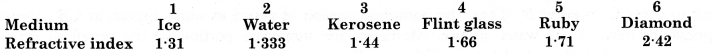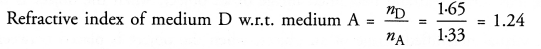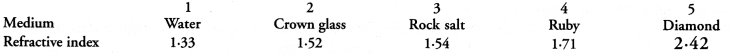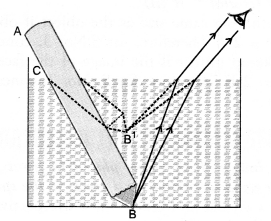## HOTS Questions for Class 10 Science Chapter 10 Light Reflection and Refraction

These Solutions are part of HOTS Questions for Class 10 Science. Here we have given HOTS Questions for Class 10 Science Chapter 10 Light Reflection and Refraction

Question 1.
You have a spherical mirror. The image of an object placed in front of the mirror is virtual. If the position of the object is changed, the image remains virtual and erect. Is the spherical mirror concave or convex ?
Since convex mirror always forms a virtual and erect image irrespective of the position of the object in front of the mirror. So the given spherical mirror is convex mirror.

More Resources

Question 2.
The following table gives the value of refractive indices of a few media.Name the medium having highest optical density and a medium having lowest optical density.
(Similar to Text Book Question, CBSE 2012, 2016)
Optical density of a medium is directly proportional to its refractive index. Therefore, diamond has the highest optical density and ice has the lowest optical density.

Question 3.
Refractive indices of kerosene, turpentine and water are 1.44, 1.47 and 1.333 respectively. Through which of these media, light travels fast ? Explain. (Similar to Text Book Question)Since, refractive index of water (1.333) is less than the refractive indices of kerosene and turpentine, so light travels faster in water than in kerosene and turpentine. Refractive indices of media A, B, C and D are given below :Question 4.
In which of these four media is the speed of light  (i) maximum and (ii) minimum ? Find refractive index of medium D w.r.t. medium A. (New CBSE Sample Paper, CBSE 2011, 2013, 2016)Since, refractive index of medium A is the least, so speed of light in medium A is maximum.
Refractive index of medium D is the highest, so the speed of light in medium D is minimum.Question 5.
The following table gives the values of refractive indices of a few mediaUse this table to give an example of (i) a medium pair so that light speeds up when it goes from one of these media to another, (ii) a medium pair so that light slows down when it goes from one of these media to another. (CBSE Sample Paper 2008)

1. Light speeds up or speed of light increases when it travels from a medium of higher refractive index to a medium of lower refractive index. Therefore, speed of light increases when it travels from crown glass or water or from rock salt to water or from diamond to water.
2. Light slows down or speed of light decreases when it travels from a medium of lower refractive index to a medium of higher refractive index. For example, when it travels from water to crown glass or from water to diamond.

Question 6.
Explain with the help of a diagram, why a pencil partly immersed in water appears to be bent at the water surface ? (CBSE (Delhi) 2008, 2011)